Deep Learning-Based Food Calorie Estimation Method in Dietary Assessment

Although food packaging comes with nutrition labels, it’s still not convenient for people to reference. Thus, scientists started to use machine learning algorithms in computer vision to help people determine the caloric value in the food they eat.

Due to the improvement in people’s standards of living, obesity rates are increasing at an alarming speed, and this is reflective to the risks in people’s health. People need to control their daily calorie intake by eating healthier foods, which is the most basic method to avoid obesity. However, although food packaging comes with nutrition (and calorie) labels, it’s still not very convenient for people to reference. Thus, scientists started to use machine learning algorithms in computer vision to help people determine the caloric value in the food they eat. During the 2015 Rework Deep Learning Summit in Boston, Google scientist Kevin Murphy presented a deep learning algorithm that was used to analyze static food image. By analyzing the composition of the food in picture, the algorithm can calculate how much calories the dish has.

This paper is trying to provide a more efficient way of estimating calories. First, it needs the top view and side view images of the food being analyzed. Then, it will use Faster R-CNN to detect the food and calibration object, after which, a GrabCur algorithm is used to determine the food’s contour. After estimating the volume of food, the authors can finally estimate the amount of calories.

Introduction

When people’s Body Mass Index (BMI) is over 30 (kg/m2), they are generally considered to be obese. High BMI can increase the risk of illnesses like heart disease . The main reason of obesity is due to the imbalance between the amount of caloric intake (consumption) and energy output (expenditure). Because of unwillingness to record and track, lack of related nutritional information or other reasons, patients often experience trouble in controlling the amount of calories they consume. There are lots of proposed methods to estimate calories based on computer vision [2, 3, 4, 5], but after the authors’ analysis, the accuracy of detection and volume estimation still need to be improved. In this paper, the main difference from other similar approaches is that it requires an input of two images, and the use Faster R-CNN to detect the object and GrabCut algorithm to obtain each food’s contour. After that, the authors can estimate each food’s volume and calories.

Material and Methods

A. Calorie Estimation Method Based On Deep Learning

This method is shown in Figure 1. As mentioned before, the process of estimating calories requires two images from top and side, and each image should include the calibration object. Here, the authors choose Faster Region-based Convolutional Neural Networks (Faster R-CNN)  to detect objects, and GrabCut algorithm  as the segmentation algorithm.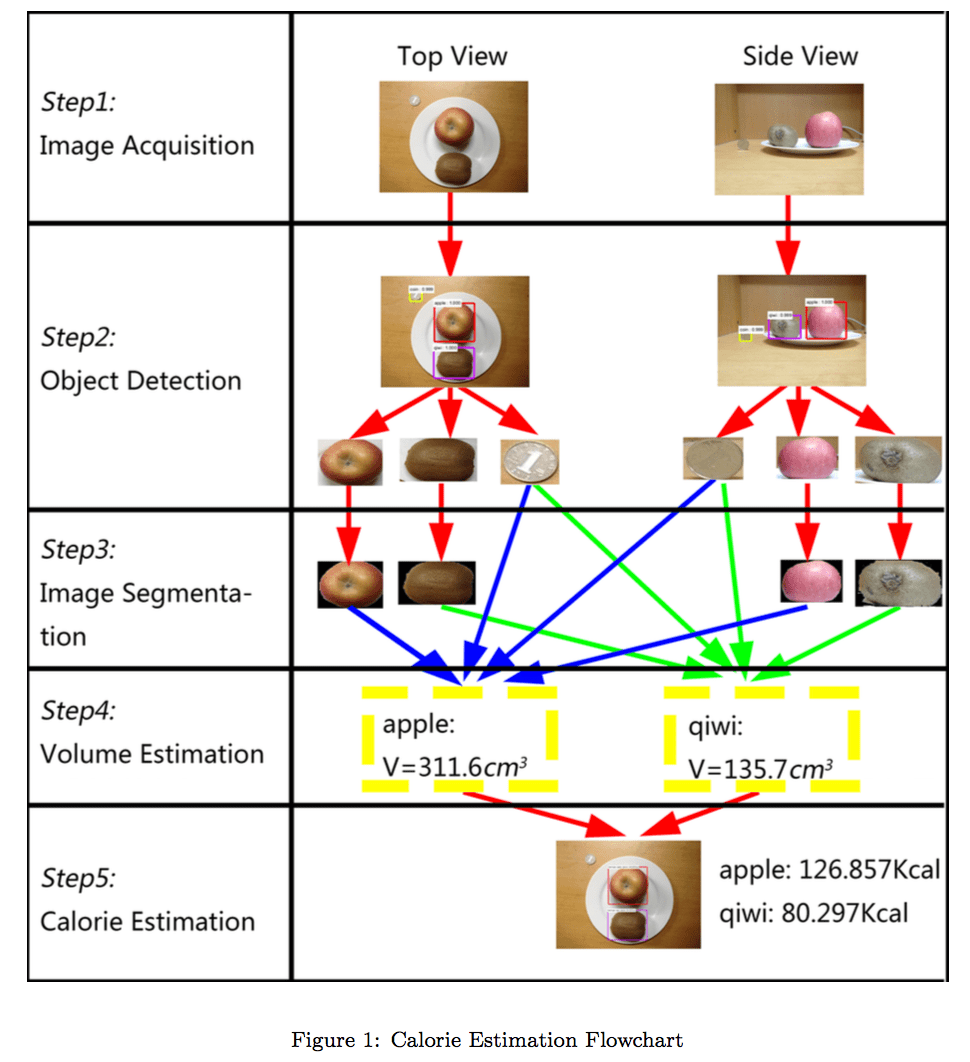B. Deep Learning Based Objection Detection

The authors chose Faster R-CNN instead of using semantic segmentation method such as Fully Convolutional Networks (FCN). Here, after the images are inputted as RGB channels, the authors can get a series of bounding boxes, which means the class if judged.

C. Image Segmentation

This process uses an image processing approach to segment each bounding box. As mentioned above, the bounding boxes around the object that GrabCut needs can be provided by Faster R-CNN. After segmentation, we can get a series of food images stored in matrix, but with the the values of the background pixels being replaced by zeros. This will leave only the foreground pixels.

D. Volume Estimation

To estimate the volume, the authors calculate the scale factors based on calibration objects. The authors use a 1 CNY coin to show the specific process of calculating the volume. The diameter of the coin is 2.5 cm, and the side view’s scale factor was calculated with Equation 1.In this equation, Ws is the width of the bounding box, Hs is the height of the bounding box. Similarly, the top view’s scale can be calculated with Equation 2.After, the authors divide the foods into three categories based on shape: ellipsoid, column, irregular. Different volume estimation formula will be selected for different types of food, according to Equation 3. HS is the height of side view PS and LkS is the number of foreground pixels in row k (k ∈ 1,2,…,HS). LMAX = max(Lk ,…,Lk ), it records the maximum number of foreground pixels in PS. ß is a compensation factor (default value = 1.0). After that, for each food type there will be a unique value.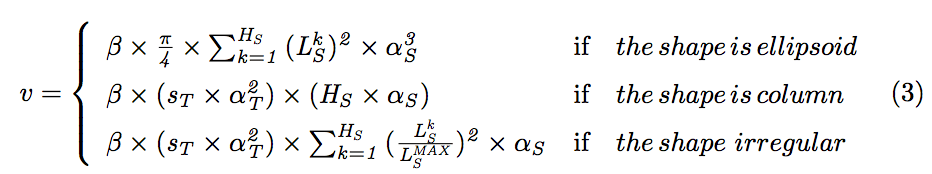E. Calorie Estimation

After estimating the volume, the next step is to estimate each food’s mass. It can be calculated in Equation 4, Where v (cm^3) represents the volume of current food, and ρ (g/cm^3) represents its density value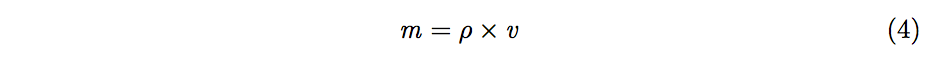Then the calorie of the food can be obtained with Equation 5.Where m(g) represents the mass of current food and c(Kcal/g) represents its calories per gram.

Results and Discussion

A. Dataset Description

In this paper, the authors use their own food dataset name ECUSTFD (downloadable on this website) ECUSTFD contains 19 kinds of food. They use a smart phone to take the required images, and each pair of images contains a top view and a side view. A 1 CNY coin is used as the calibration object. Additionally, for each image in ECUSTFD, they provide annotations, volume and mass records.

B. Object Detection Experiment

The author use a comparison experiment to choose the object detection algorithm. The numbers of training images and testing images are shown in Figure 2. Average Precision was used to evaluate the object detection results. In the test set, Faster R-CNN achieves 93.0% while Exemplar SVM achieves 75.9%.C. Food Calorie Estimation Experiment

ß (compensation factor) in Equation 3 can be calculated with Equation 6, where k is the food type, and N is the number of volume estimation.p in Equation 4 can be calculated with Equation 7.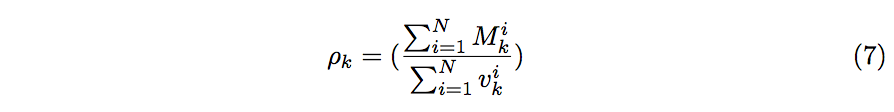After that, the authors give the shape definition, estimation images number, ß, ρ of each food in Table 1.Then, by using the images from the test set, the results can be shown in Table 2.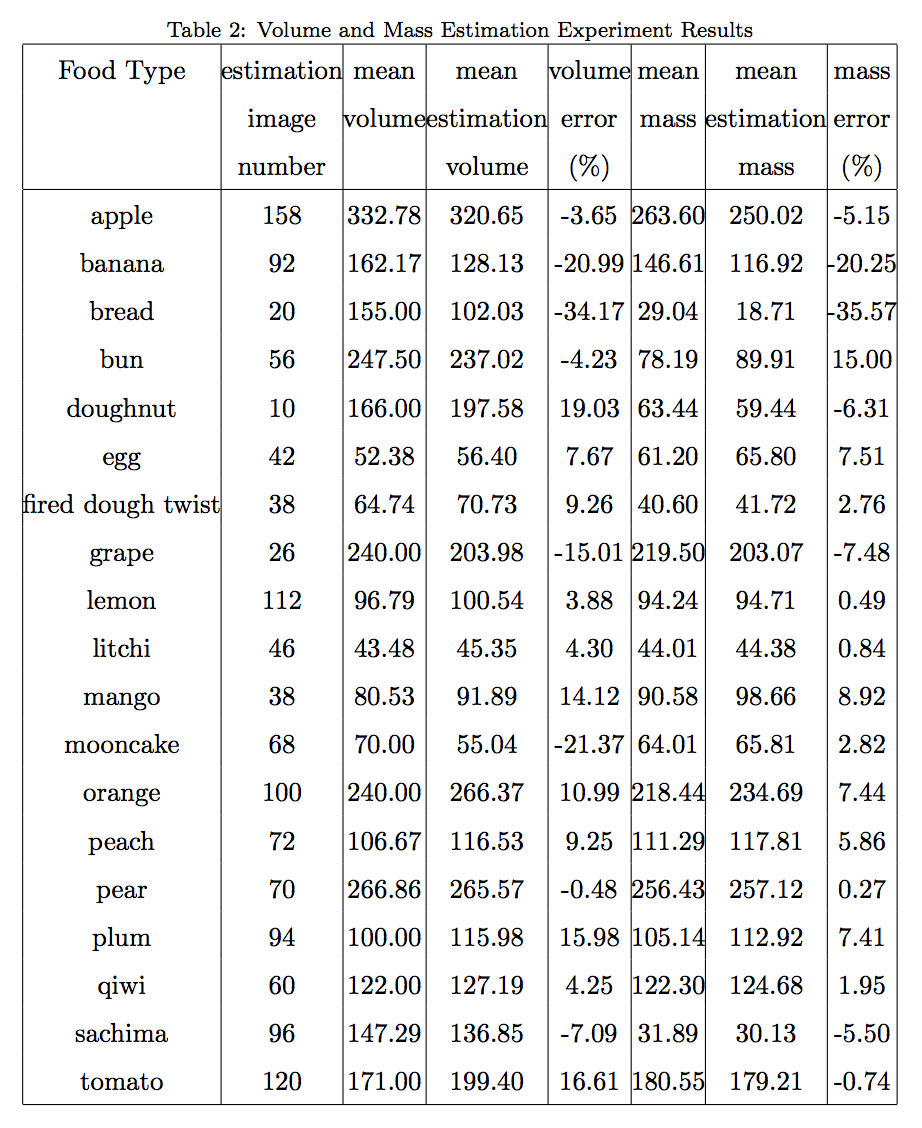The authors use mean volume error to evaluate volume estimation results. The definition of mean volume error is as shown in Equation 8, where food type is i, 2Ni is the number of images Faster R-CNN recognizes correctly.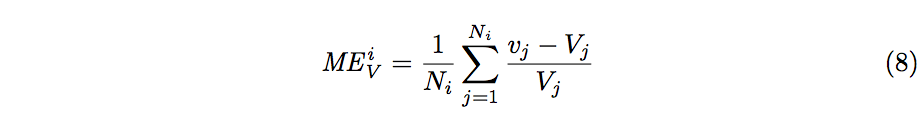The definition of mean mass error is in Equation 9.For the results in Table 2, we see that most types of food’s estimation results are closer to reference real values. Other than banana, bread, and mooncake, the mean error between estimation volume and true volume does not exceed ±20%. Even if the drainage method is not that accurate, but the estimation method can be accepted.

Conclusion

This paper gives us a calorie estimation method, and the results of the experiments show promise.

Since the images are taken from smartphones, and the image processing methods used here are well-developed, this proposed method can be easily integrated into health apps as an engineering solution. Nevertheless, from a research perspective, I think this paper has two limitations. First, there is no comparison with prior work. The authors did provide a literature review in the introduction, but I think they should have compared their results with the results in those prior work. If this approach can achieve a better performance, then we can say that this paper provides a more effective way. Unfortunately we cant’s say that, because the author didn’t provide a series of comparison experiment. Secondly, I am not sure if the dataset is accurate or big enough. The authors just say that they take the images from a smartphone, but they didn’t tell whether there is a standard to collect the images. Like the light intensity, and the number of pixels. Besides, in Table 2 we can see that the mean error is still large, which indicates that there is some room to make the mean error much smaller.

Reference

 W. Zheng, D. F. Mclerran, B. Rolland, X. Zhang, M. Inoue, K. Matsuo, J. He, P. C. Gupta, K. Ramadas, S. Tsugane, Association between body- mass index and risk of death in more than 1 million asians, New England Journal of Medicine 364 (8) (2011) 719–29.
 W. Jia, H. C. Chen, Y. Yue, Z. Li, J. Fernstrom, Y. Bai, C. Li, M. Sun, Accuracy of food portion size estimation from digital pictures acquired by a chest-worn camera., Public Health Nutrition 17 (8) (2014) 1671–81.
 Z. Guodong, Q. Longhua, Z. Qiaoming, Determination of food portion size by image processing, 2008, pp. 119–128.
 Y. Bai, C. Li, Y. Yue, W. Jia, J. Li, Z. H. Mao, M. Sun, Designing a wearable computer for lifestyle evaluation., in: Bioengineering Conference, 2012, pp. 93–94.
 P. Pouladzadeh, P. Kuhad, S. V. B. Peddi, A. Yassine, S. Shirmohammadi, Mobile cloud based food calorie measurement (2014) 1–6.
 S. Ren, K. He, R. Girshick, J. Sun, Faster r-cnn: Towards real-time object detection with region proposal networks, in: Advances in neural informa- tion processing systems, 2015, pp. 91–99.
 C. Rother, V. Kolmogorov, A. Blake, Grabcut: Interactive foreground extraction using iterated graph cuts, in: ACM transactions on graphics (TOG), Vol. 23, ACM, 2004, pp. 309–314.

Author: Shixin Gu | Editor: Joni Chung | Localized by Synced Global Team: Xiang Chen